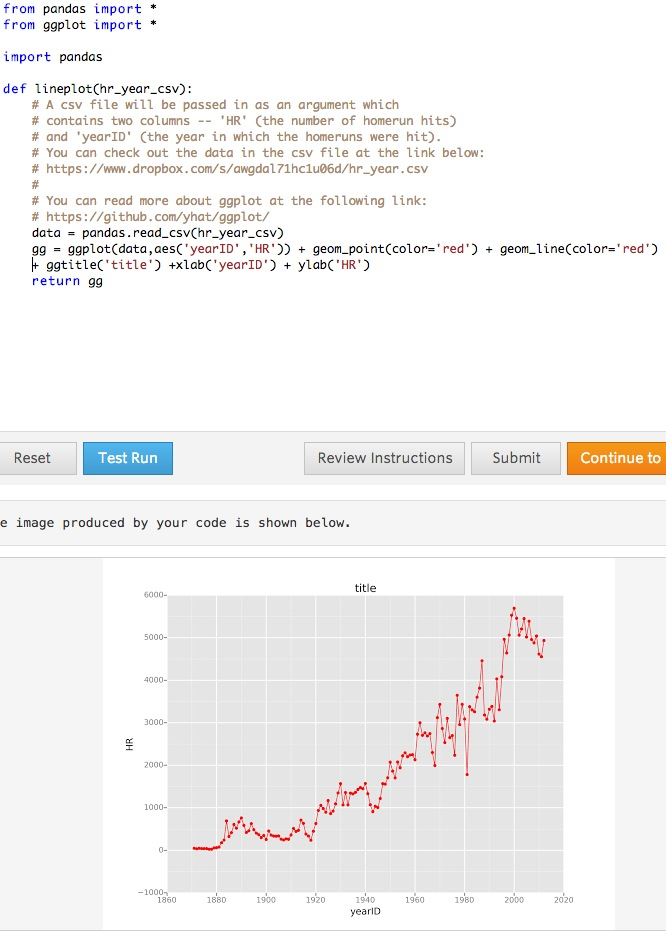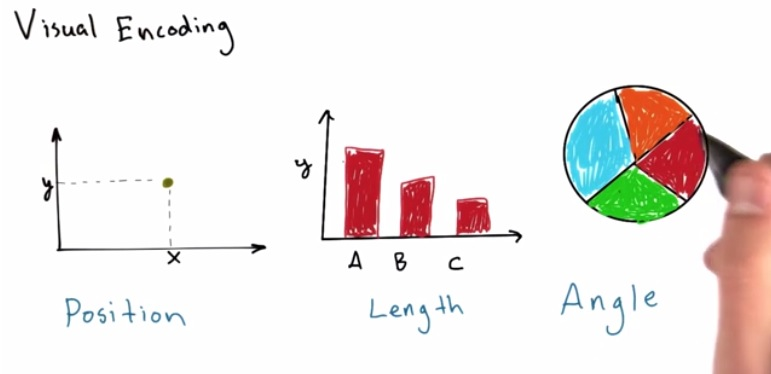• There are various ways to represent our visuals
• Position. This are effectively space-saved in our visualization. Rather than many data, we represent our data as just one dot. When too many dot exists, it's important to take into account, what can differentiate each dots. dots also useful if we want to find outliers, mean, middle, etc.
• Length. It's to represent each data sample in terms of intensity. One use example is bar chart
• Angle. One example is pie chart. This is to represent as data as whole. The disadvantage is people can't  differentiate small angle (25 vs 26 degrees). So make sure that our each of our pie doesn't have small difference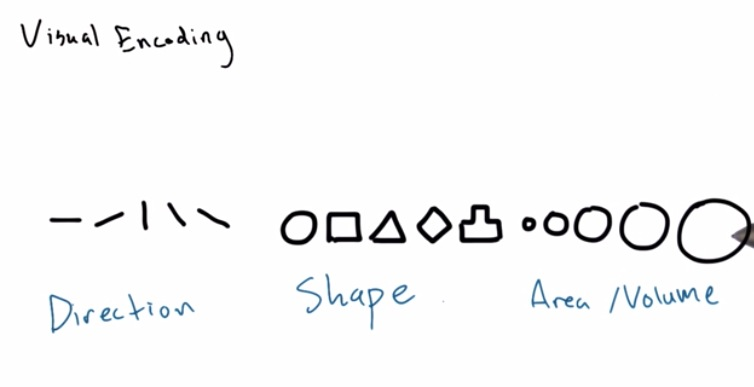• Direction. Also acts like pie chart. We can visualize if the data actually increase/decrease/flat. But also have poor implementation if we don't know which negative/positive
• Shape. This can differentiate our data types in points. Different two groups represent two different shape (clustering).
• Area/Volume. Like a bar chart, intensity represented by size. We can circling our data,(may be from Gaussian distribution) and try to find the outliers.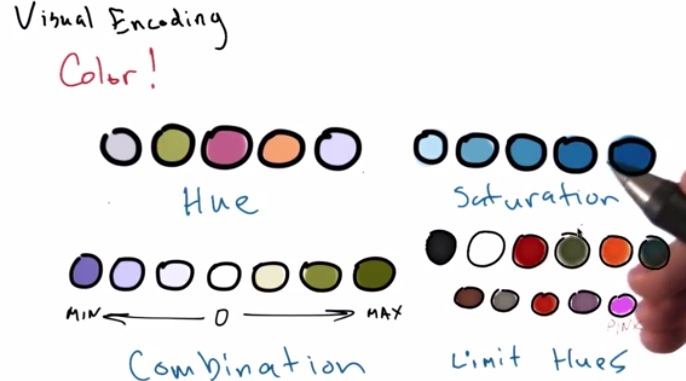• Hue is what we called color. We can use hue to differentiate different type of groups
• Saturation. Use it to make level of intensity between your data.
• Combination. Useful when we make intensity on two  different types of data. For example, intensity towards negative, or towards positive
• Keep in mind to limit hues to max 5 different color. Use it like 12 colors will lose its meaning and make it harder to visualize the data.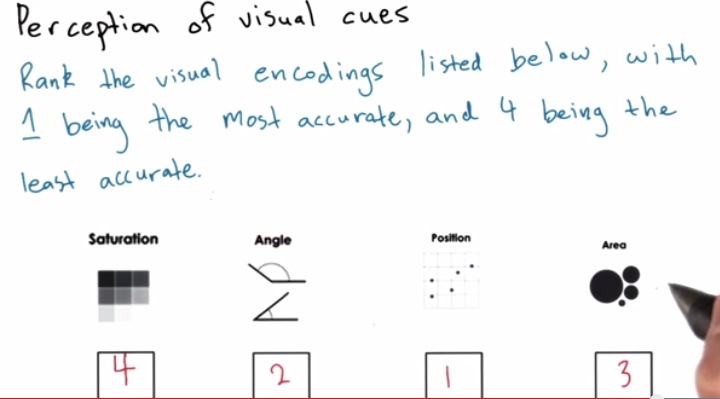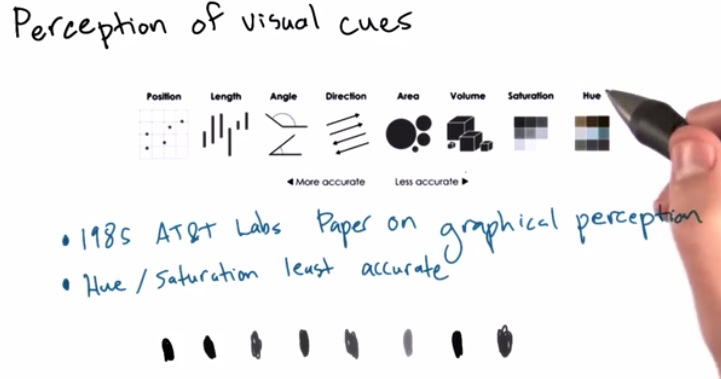• This is rank based on research
• hue and saturation may inaccurate because it makes people harder to perceive what are the data that you're trying to represent
• For example, saturation of a gray would makes people harder to differentiate the color. What are the different level of intensity represent different size of a data
• It not acts like a definitive rules but rather as a guide.• ggplot working nicely with our pandas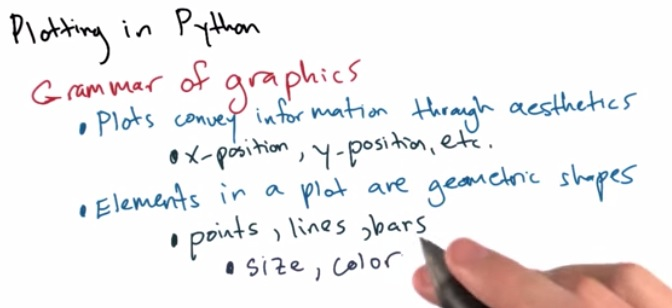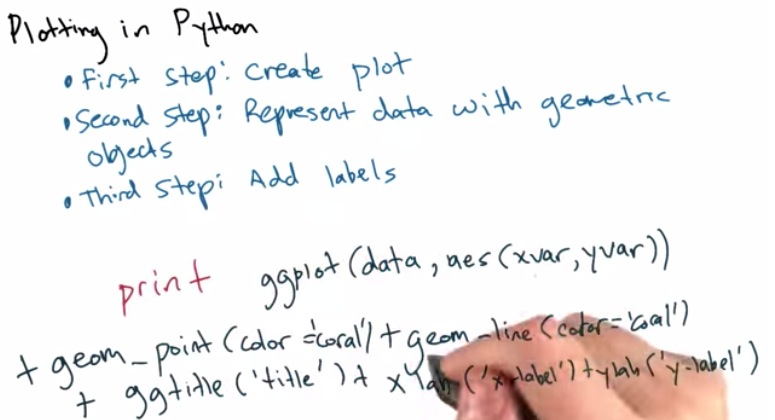1. Create ggplot. This will take as an input;
1. data: our pandas dataframe
2. xvar,yvar: 1st = x-axis, 2nd=y-axis. xvar and yvar are the two column name that we want to fetch from our dataframe
2. Create point or even line to connect the point
1. take color as an input, for point and geom line
3. Create the label
1. Sepecify the title(ggtitile) as well as the label for x-axis and y-axis